# Acta Universitatis Danubius. Œconomica, Vol 12, No 5 (2016)

An Analysis of a Duopoly with Incomplete Information

Cătălin Angelo Ioan1, Gina Ioan2

Abstract: In this paper we analyze the case of two firms A and B, each competing with the other under conditions of incomplete information. Thre analysed cases are: company A assume one of the following roles: either ignore the firm B or leading production or leading price acting as if B knows its intention and firm B acts either ignoring company A, or as satellite of production or satellite of price acting as if A knows its intention.

1. Introduction

The duopoly is a market situation where there are two suppliers of a good unsubstituted and a sufficient number of consumers.

Considering below, two competitors A and B which produce the same normal good, we propose analyzing their activity in response to the work of each other company.

Each of them, when it set the production level and the selling price will cover the production and price of the other company. If one of the two firms will set price or quantity produced first, the other adjusting for it, it will be called price leader or leader of production respectively, the second company called the satellite price, or satellite production respectively.

The approach of the following considerations will be that company A will assume a role: either ignore the firm B or leading production or leading price acting as if B knows its intention and firm B acts either ignoring company A, or as satellite of production or satellite of price acting as if A knows its intention.

Let therefore two producers, the demand inverse function: p(Q)=a-bQ, a,b0, total costs of A and B being TCA(Q)=Q+, TCB(Q)=Q+, ,,,0 where Q is the production. We have now: MCA= and MCB= - marginal costs of each firm.

2. The Case of Cournot Equilibrium

In this case, both companies A and B act independently of each other and at the same time.

Considering the profits of both firms, we have: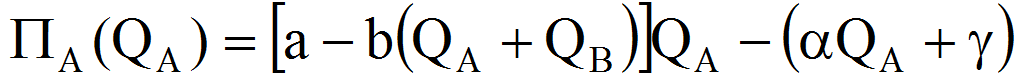=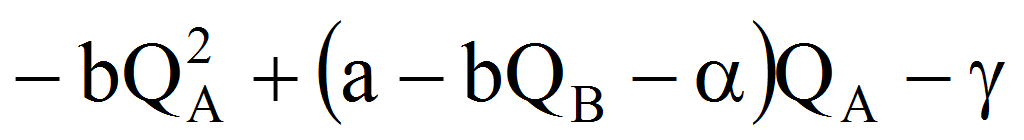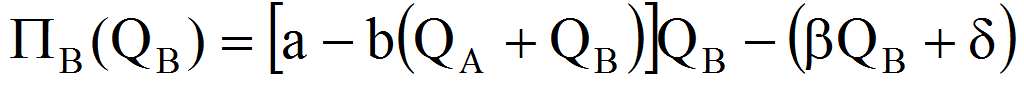=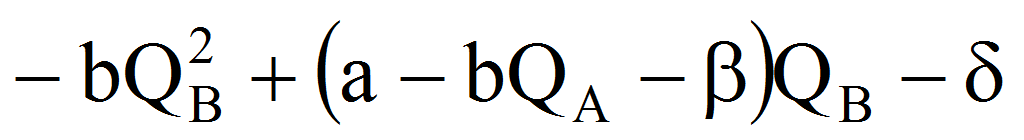In order to maximize the profits, we must have: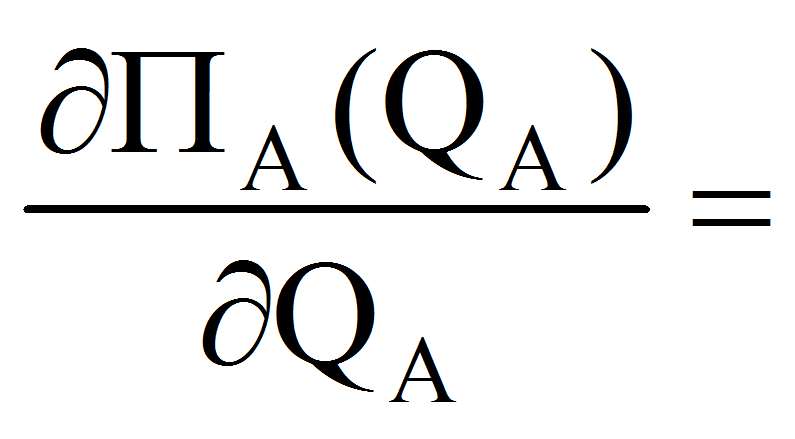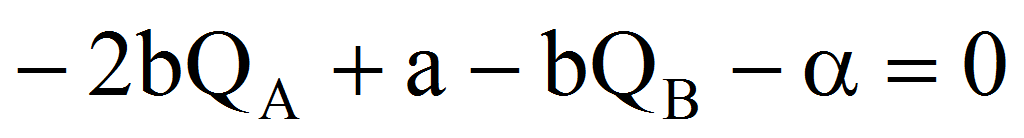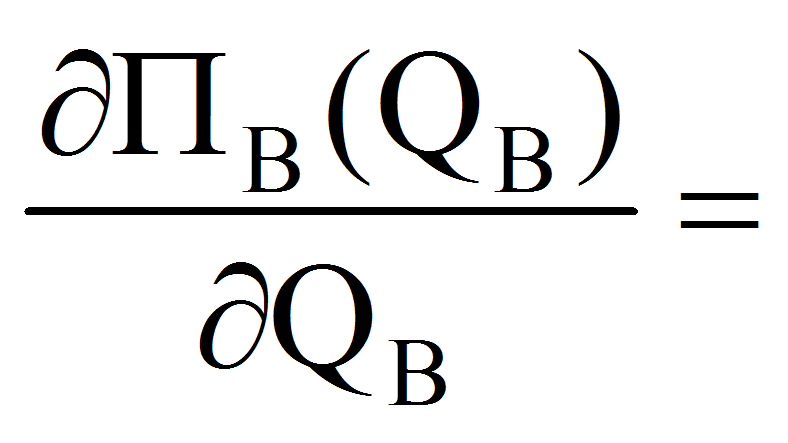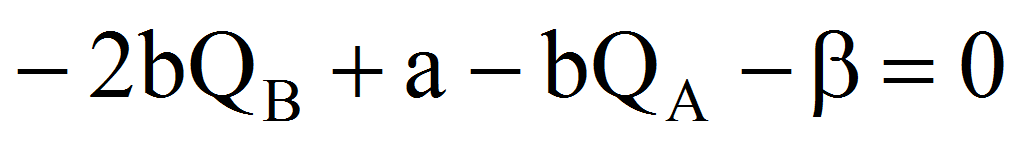from where (noting with C from Cournot):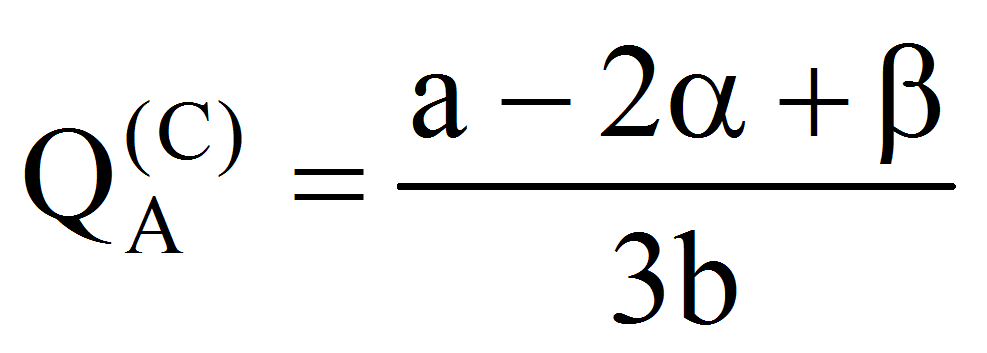,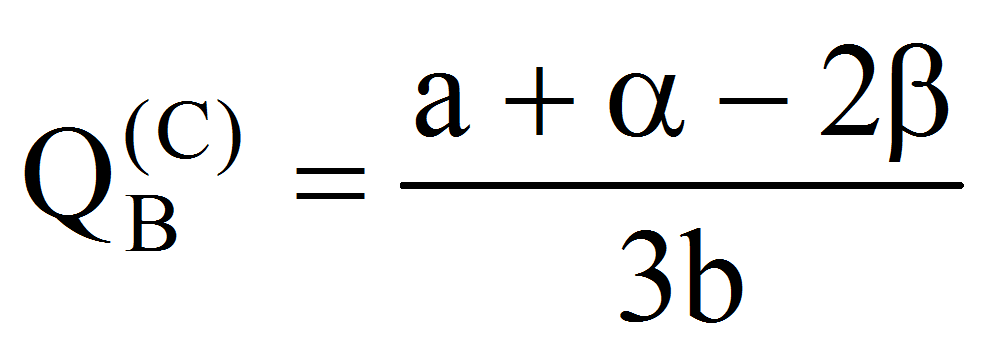The price will be: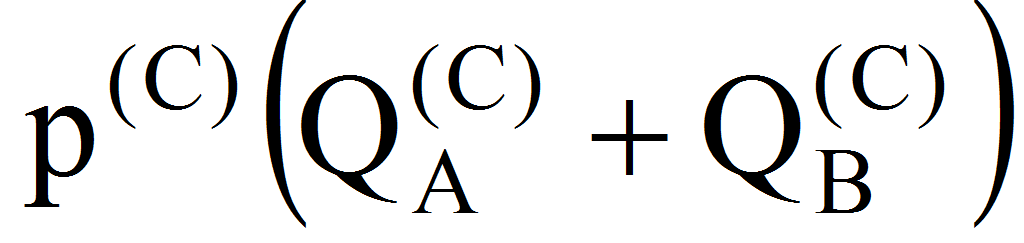=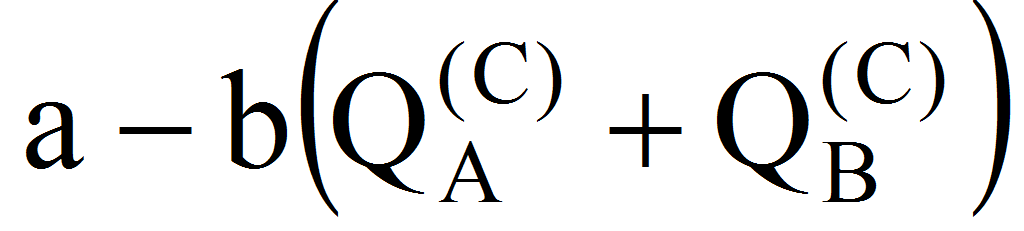=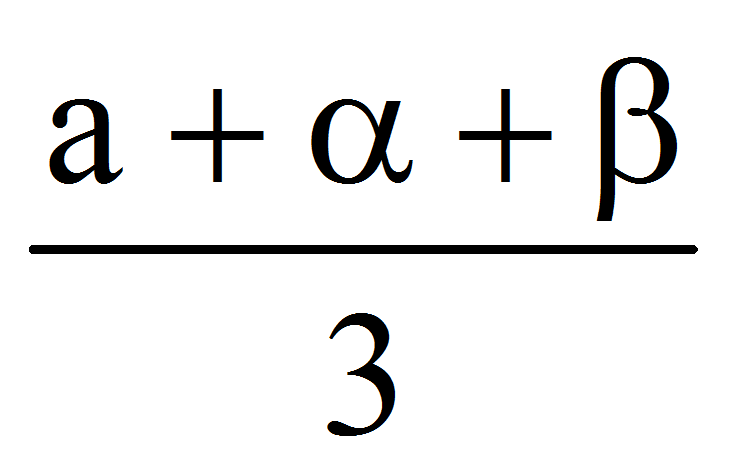and the profits: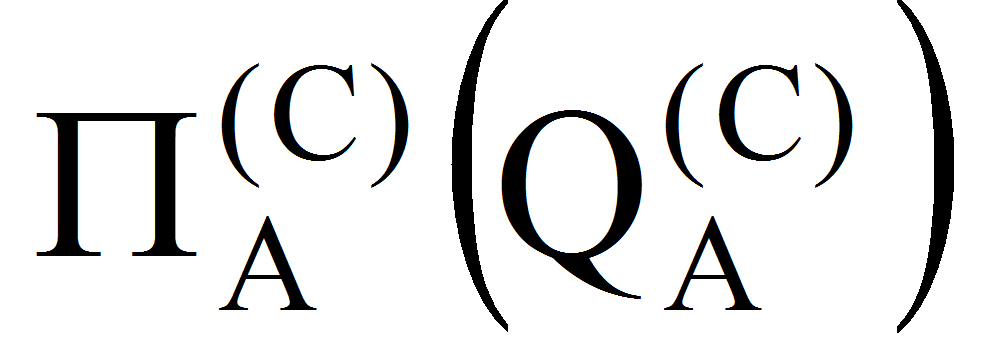=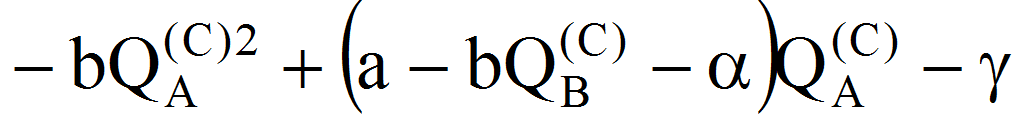=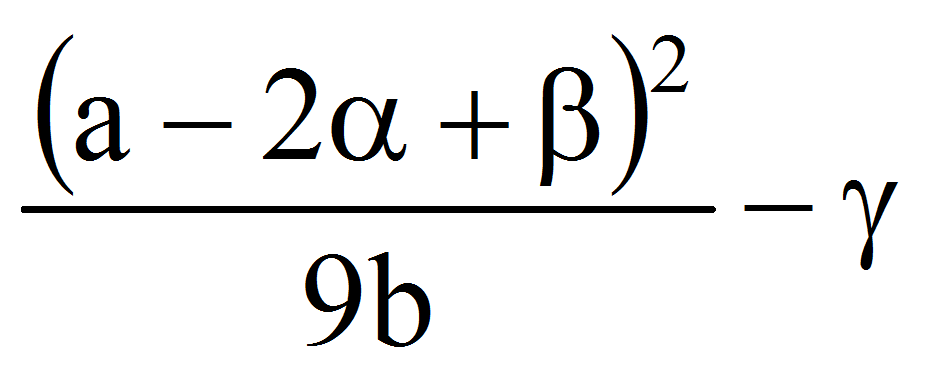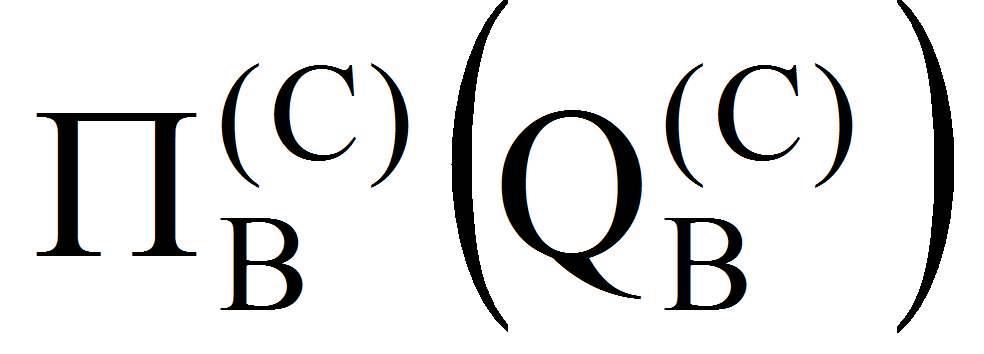=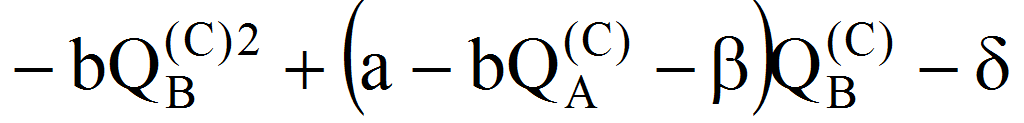=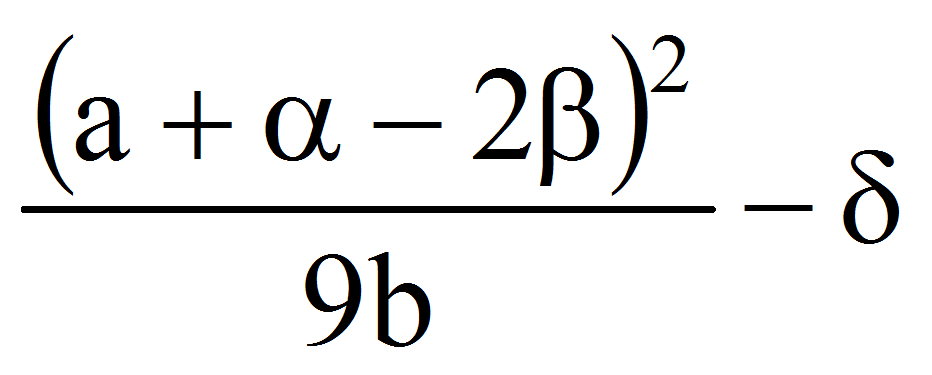We have now (noting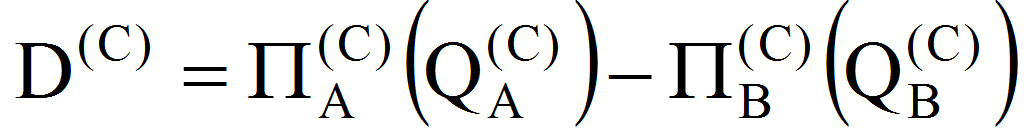- the difference between two profits):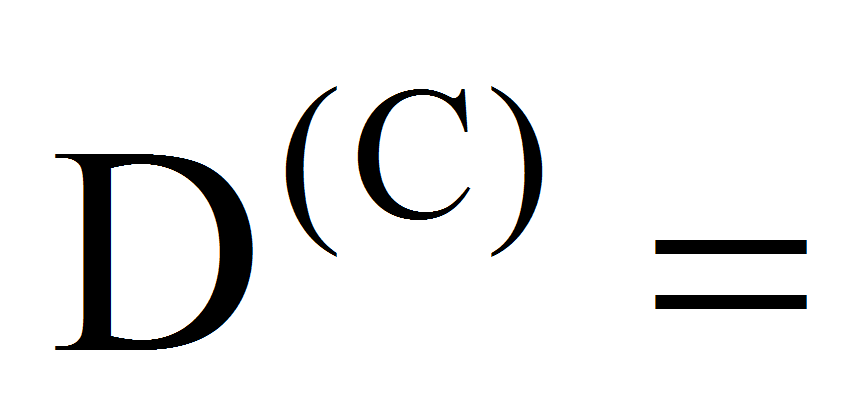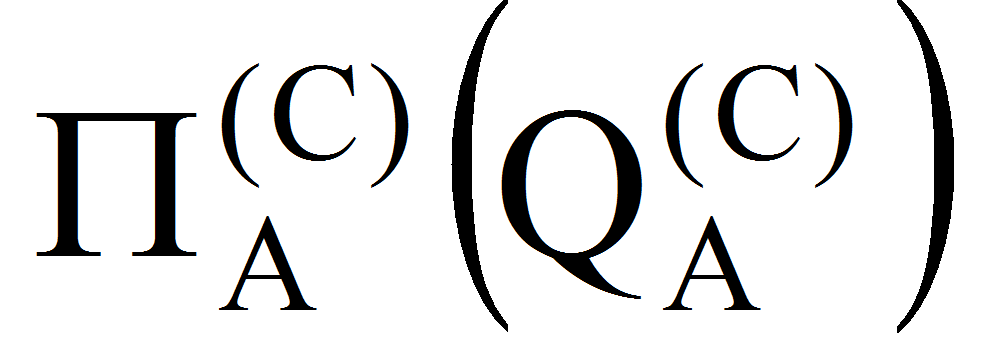-=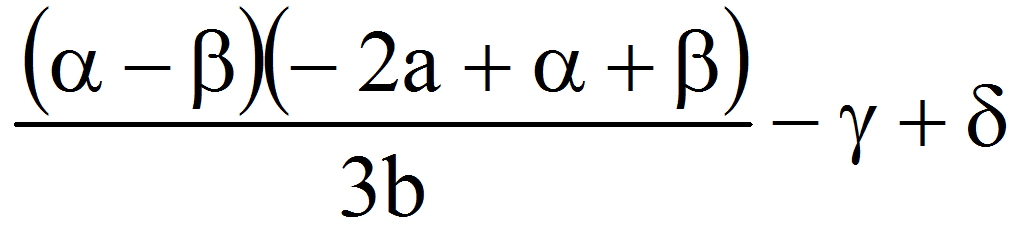3. The Case of Stackelberg Production Leader and Satellite in Terms of Production

In this case, the company A assumes the role of production leader and company B recognizes this.

Because the company A is a leader of production, it will produce QA units of a good. The company B will adjust production after A, in response to its leadership, producing QB=f(QA) units of good (f - the reaction function). Because the selling price depends on the total quantity of products reached the market, we have p=p(QA+QB) – the price per unit of good.

On the other hand, the company A must establish a level of production depending on the reaction of firm B, because it will determine through the production realized the selling price of the product. Similarly, the company B will adjust its production levels according to A, because at a higher or lower level, the price will change and therefore profit of the company ().

Let therefore, the profit of the production leader: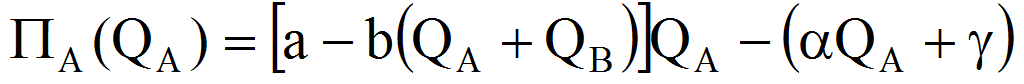=Since QB=f(QA) we have: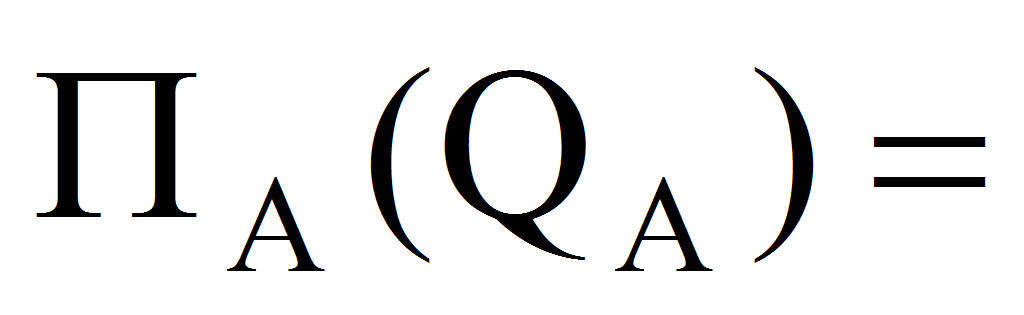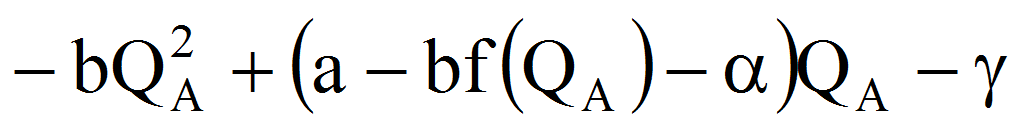Consider also the profit of the satellite:=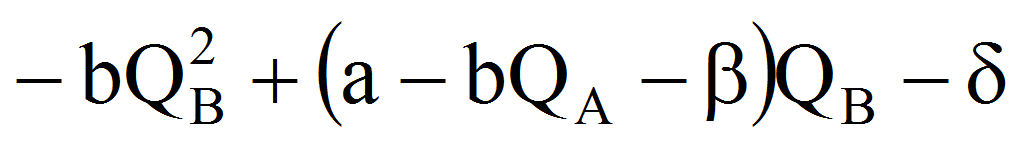The extreme condition for the profit of B is: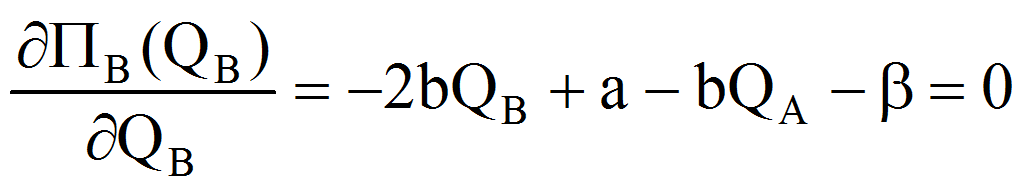therefore: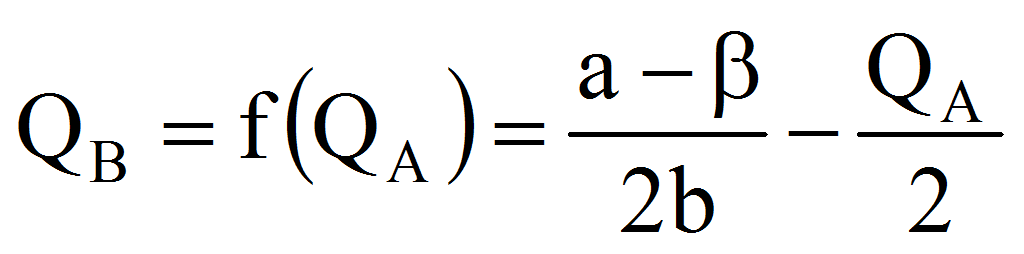Now the profit of A will become: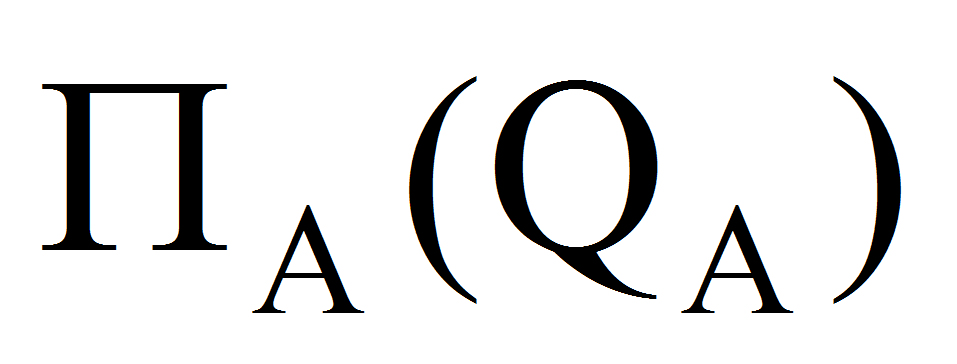=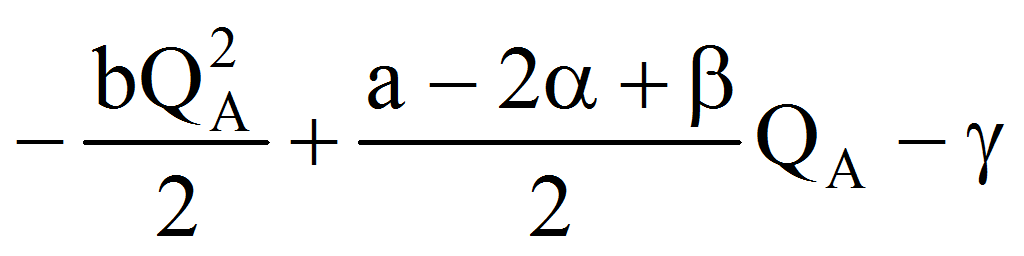and the condition for maximizing: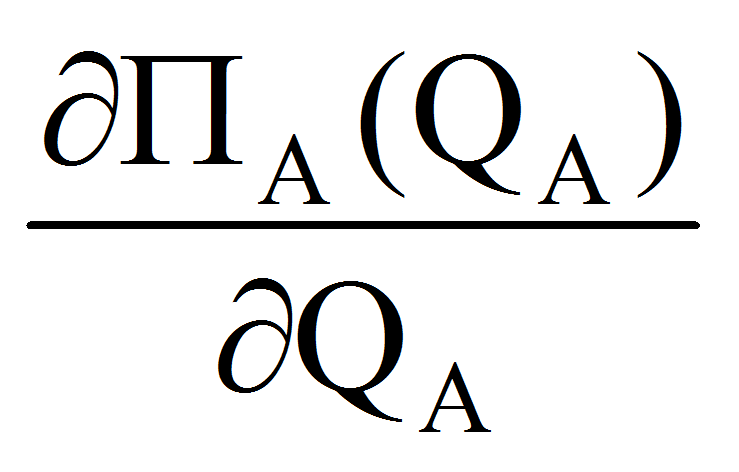=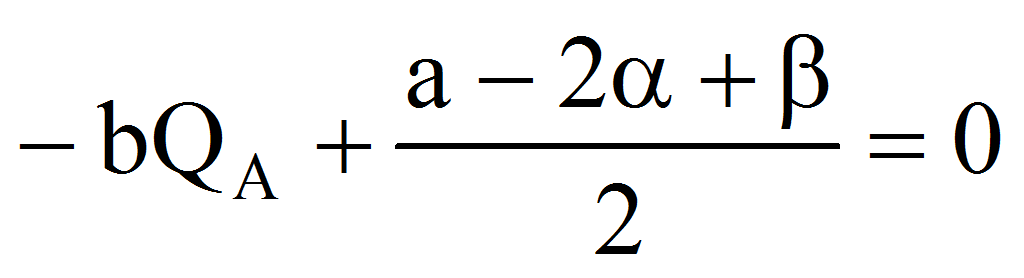therefore (noting with PL from production leader):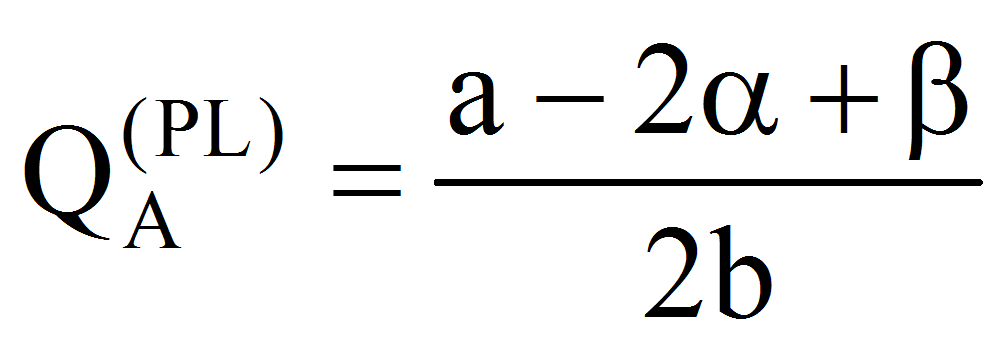,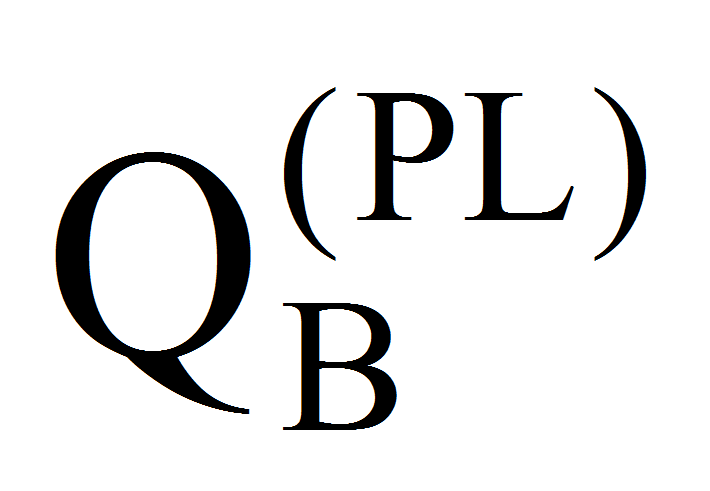=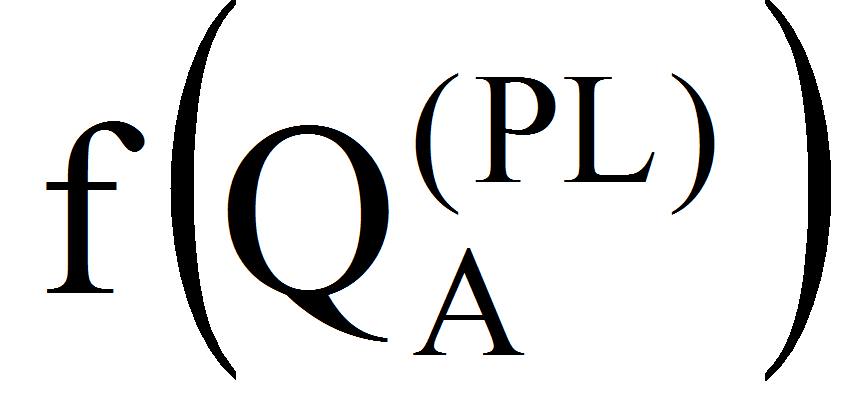=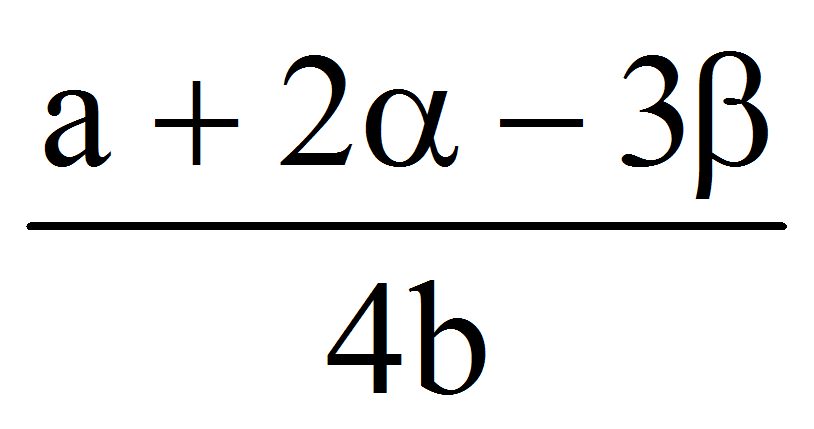and the price will be: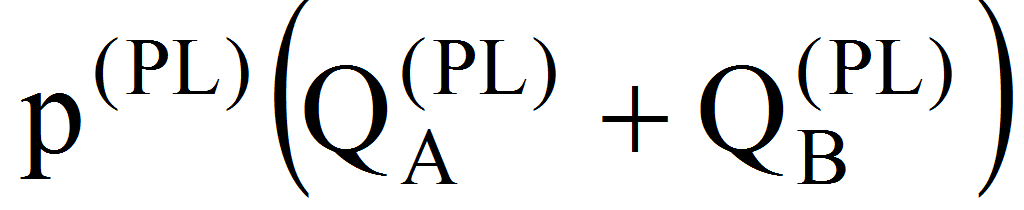=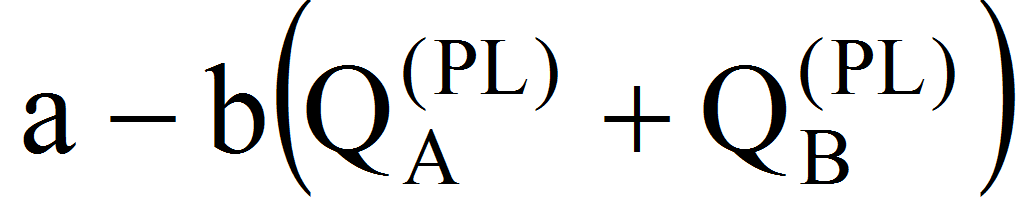=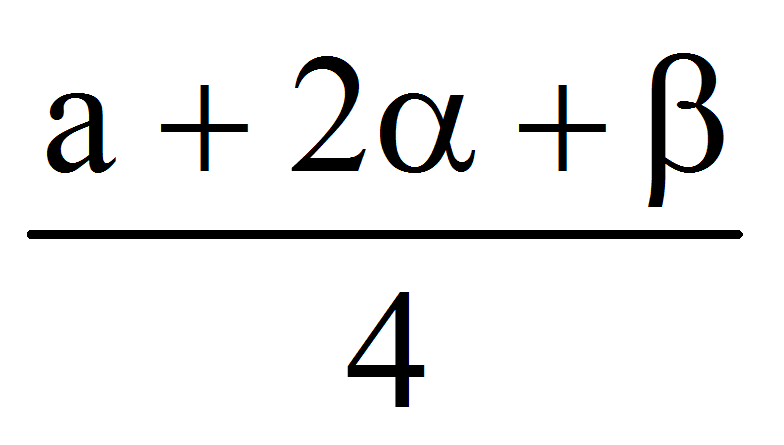The profits of both firms A and B are: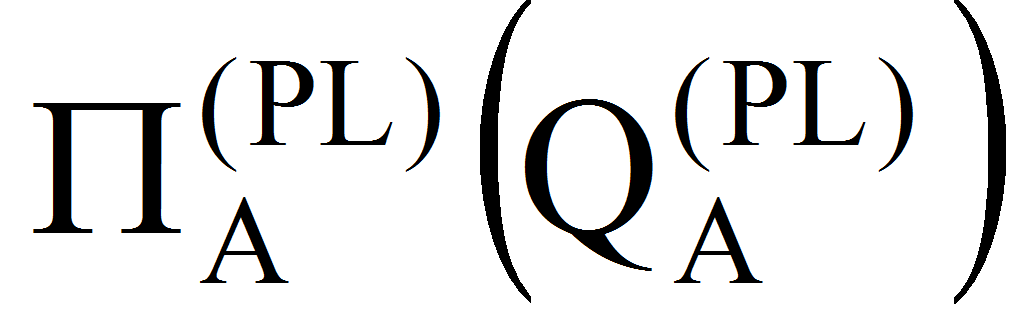=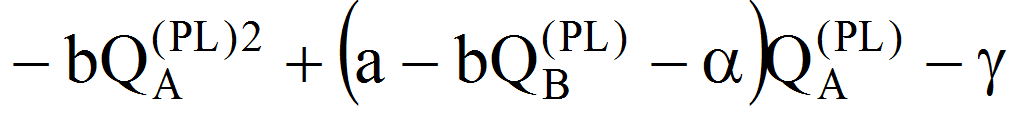=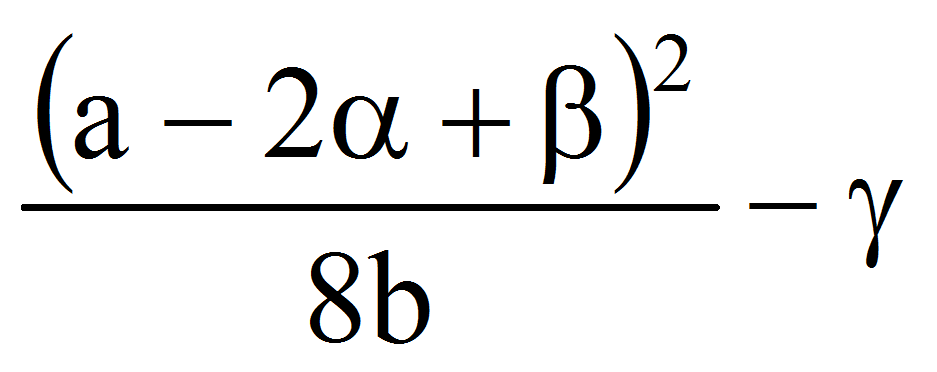respectively: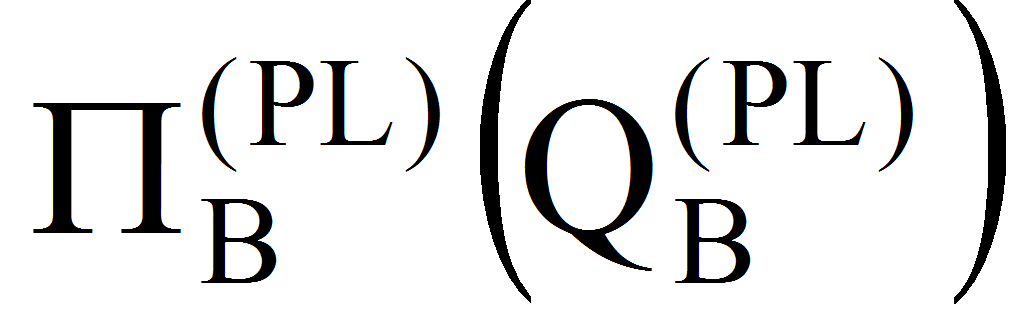=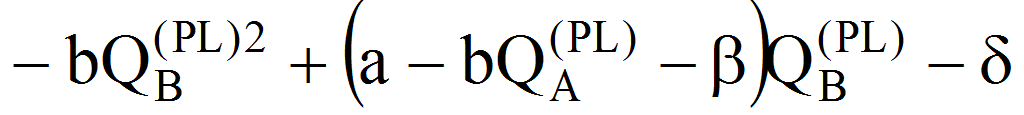=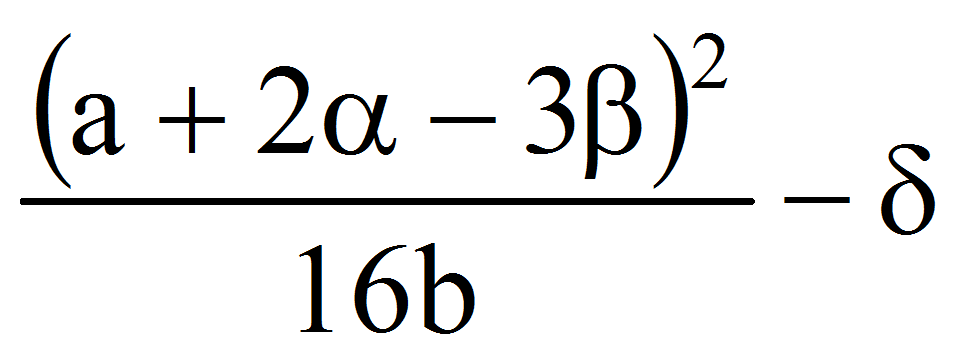Because A assumes that it is leader of production, we must have: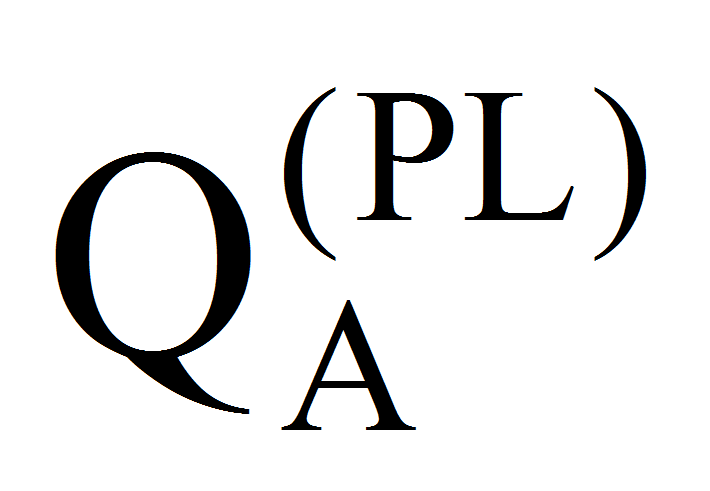that is: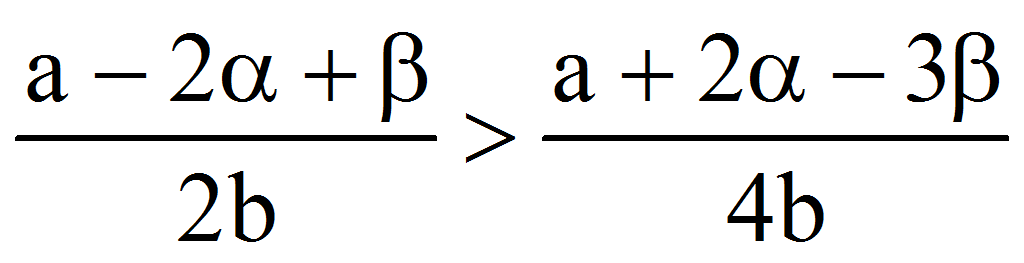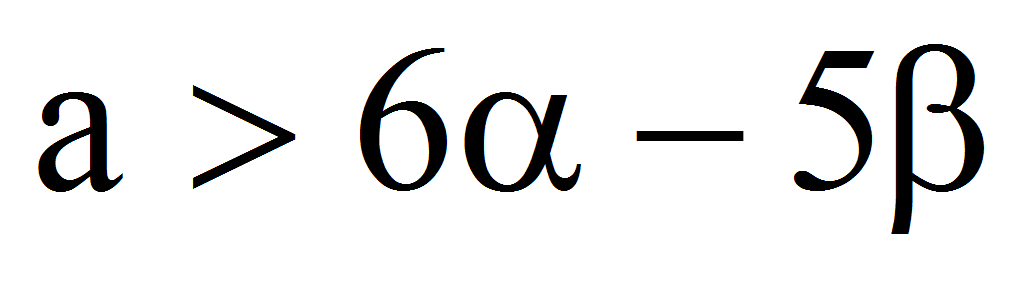.

We have now (noting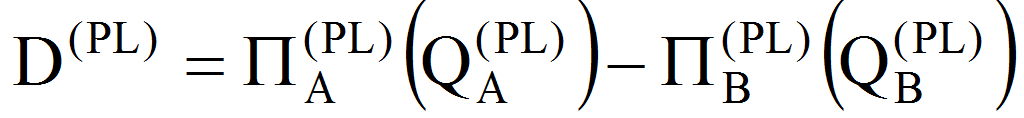- the difference between two profits):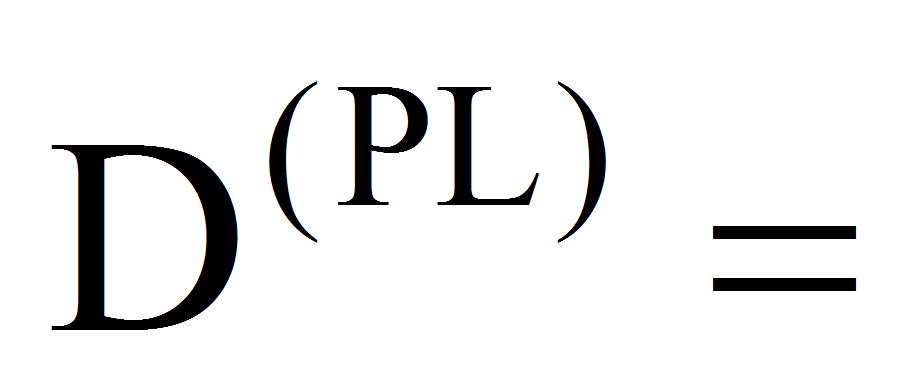-=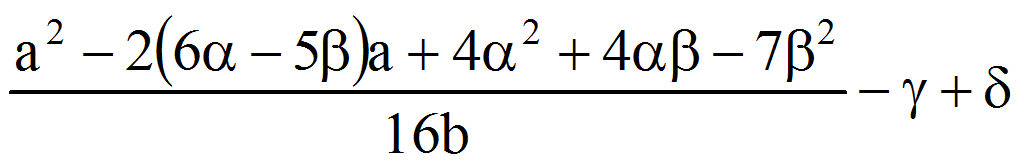4. The Case of Stackelberg Price Leader and Satellite in Terms of Price

In this case, the company A assumes the role of price leader and company B recognizes this.

It is obvious that, regardless of the satellite firm behavior, the final sale price will be the same for the two companies, otherwise the demand being moving to the company with the lowest price.

Let the price being p0 – fixed in the final.

The profit functions of the two companies are therefore: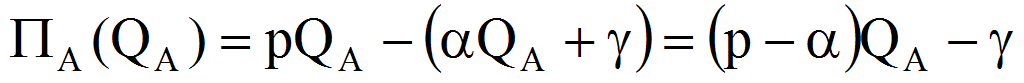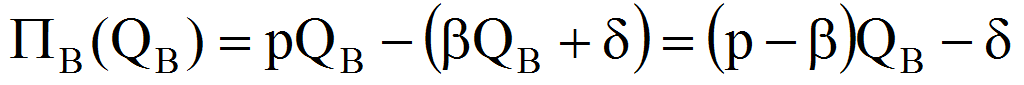From these formulas we have the necessary condition that p, p (otherwise0 or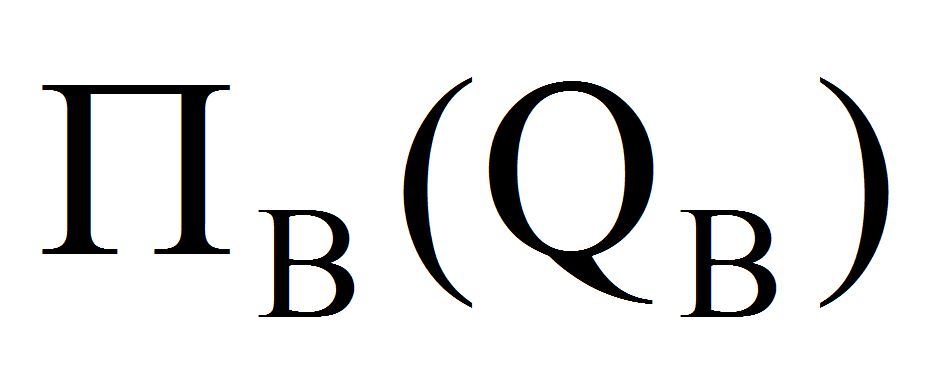0).

Because both profit functions are of first degree in Q and p-0, p-0 they reach the maximum at infinity.

In this case (with linear total cost of B) the production of B will be, for the moment, indeterminate, let say QB=q0.

Meanwhile, the company leadership is aware that setting a selling price p will lead a production QB of the satellite firm, so in terms of a demand curve Q=Q(p) its offer will be restricted to QA(p)=Q-QB=Q(p)-q=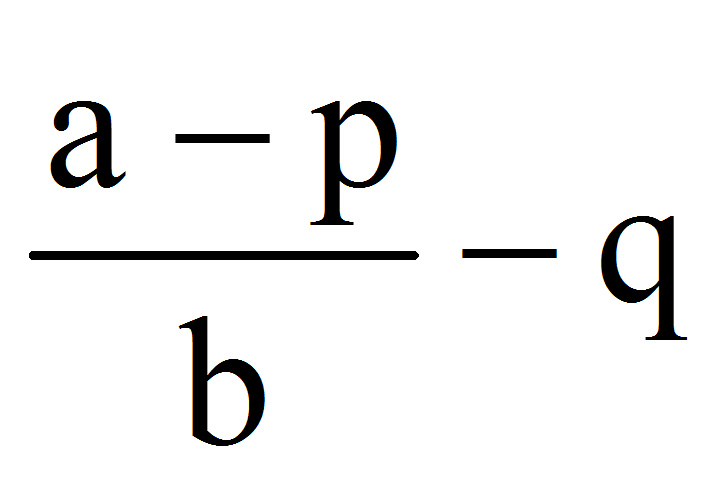=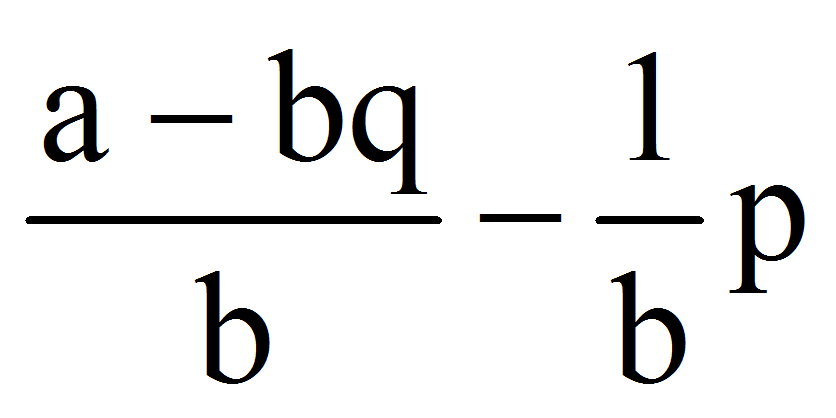. Because QA(p)0 we must have: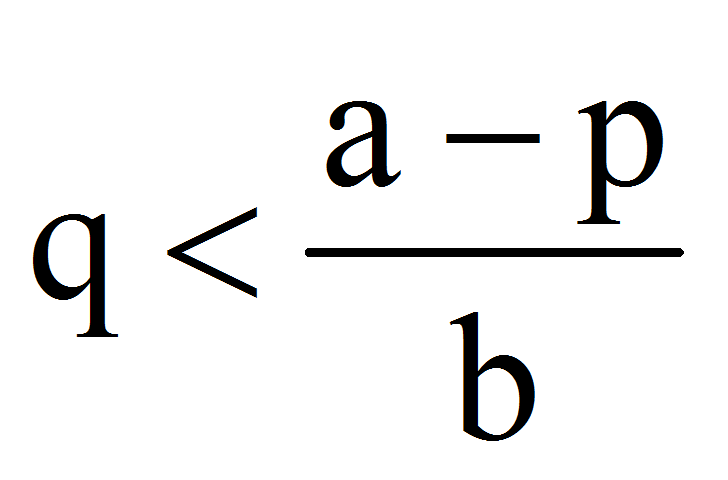. If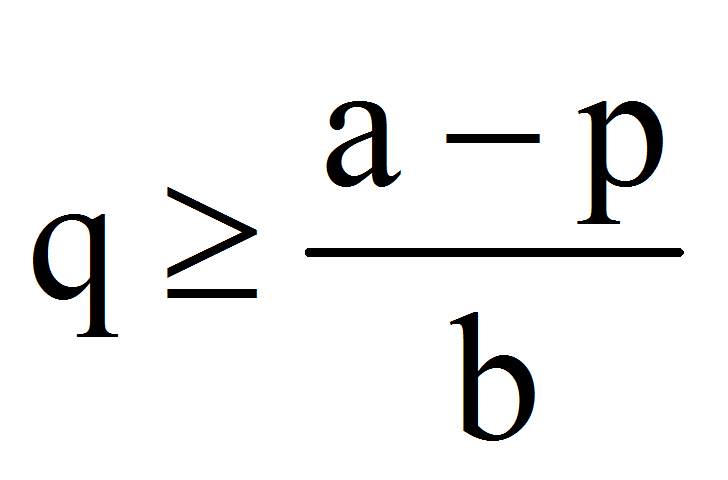the production of A: QA(p)0, therefore A gives up at the price leader role.

Its profit function becomes: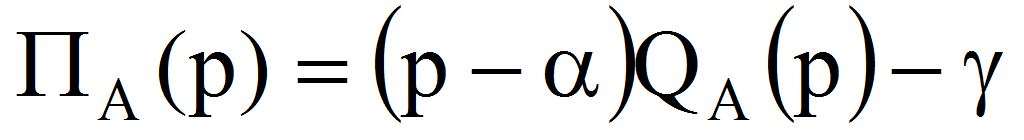=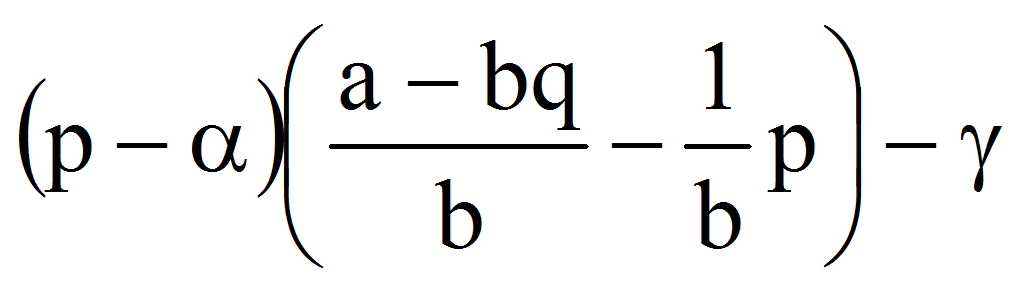=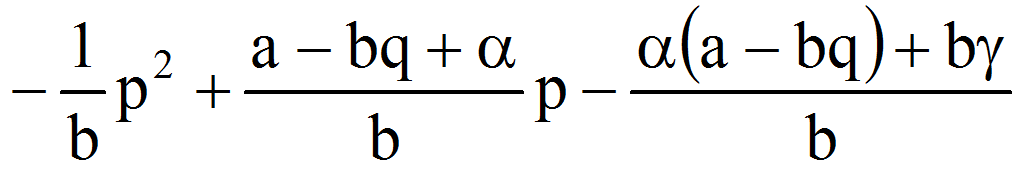The profit maximization condition of A is therefore: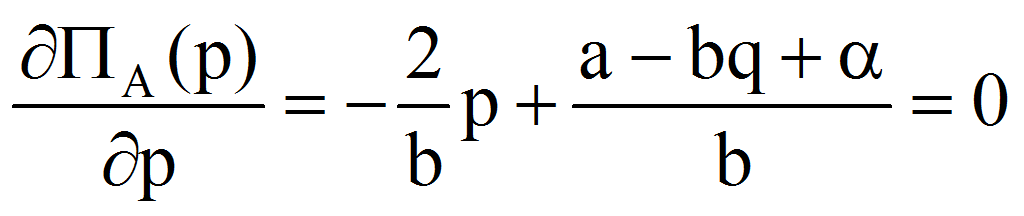from where (noting with PP from production price):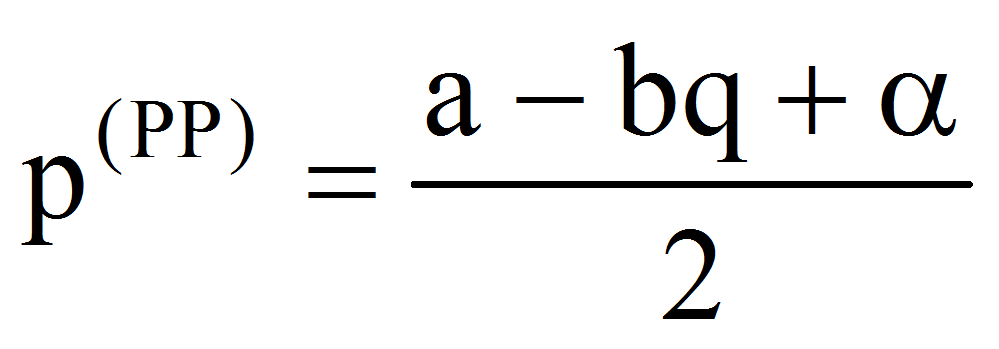From this relation, because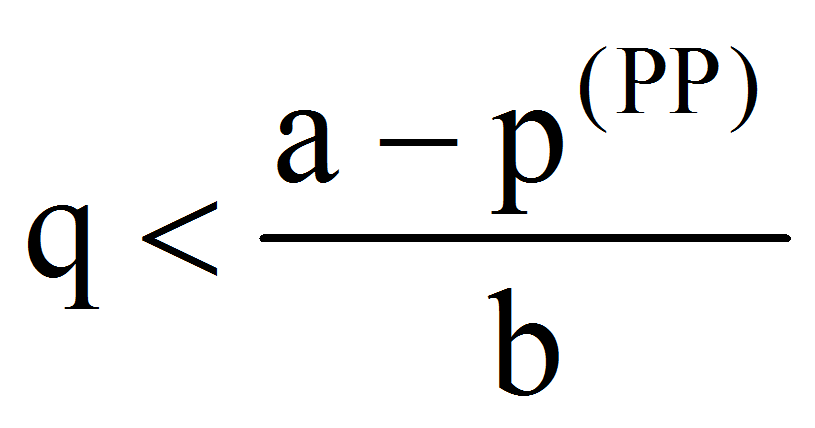we have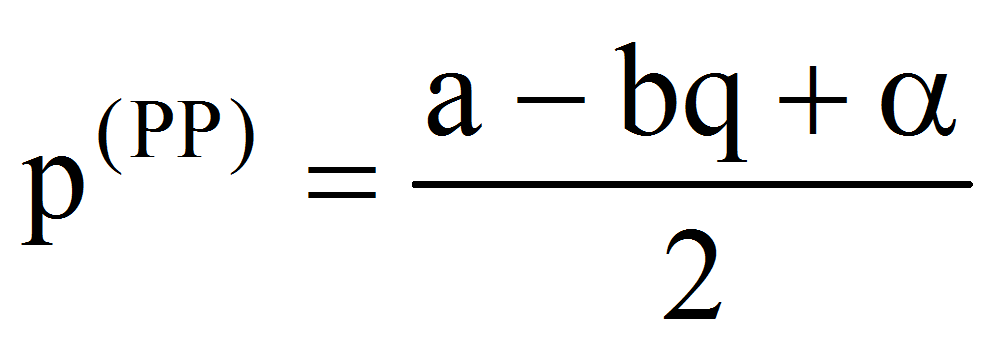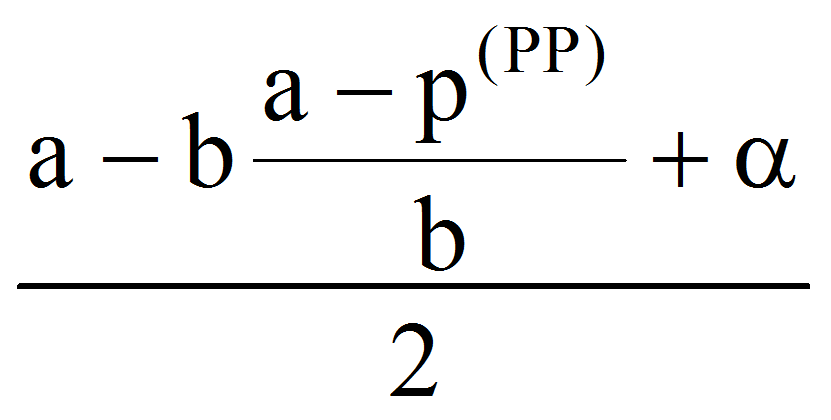=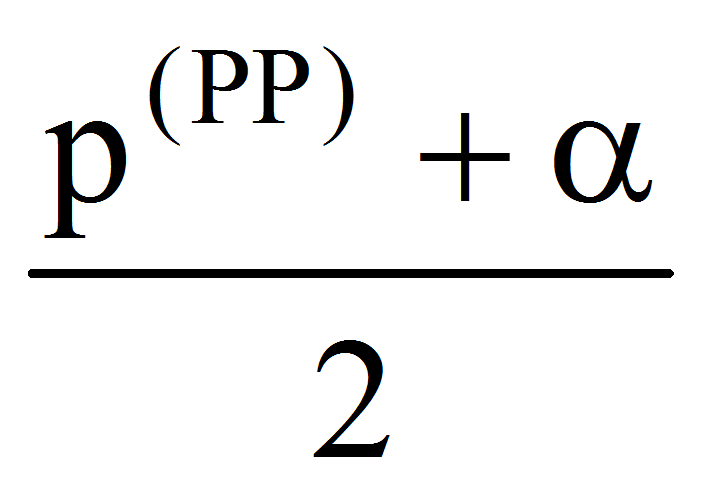that is: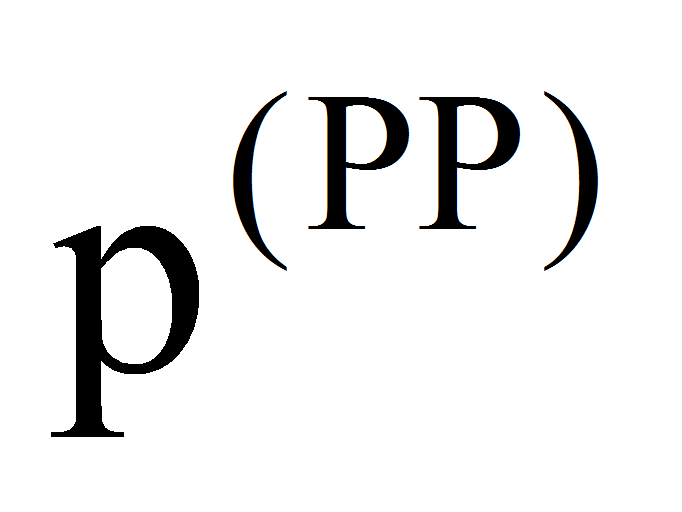 which satisfies the initial condition on the price.

Finally: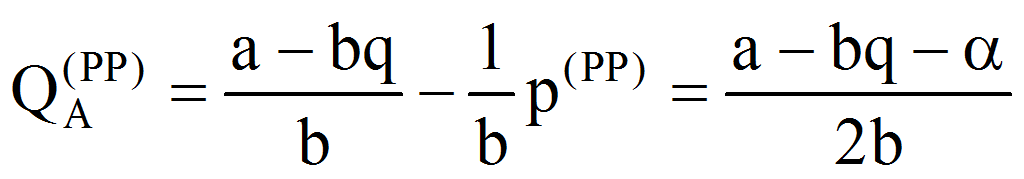,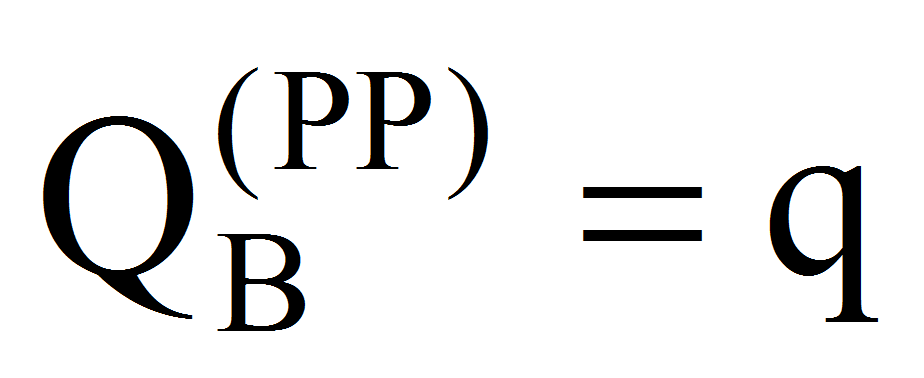The profits of both firms A and B are: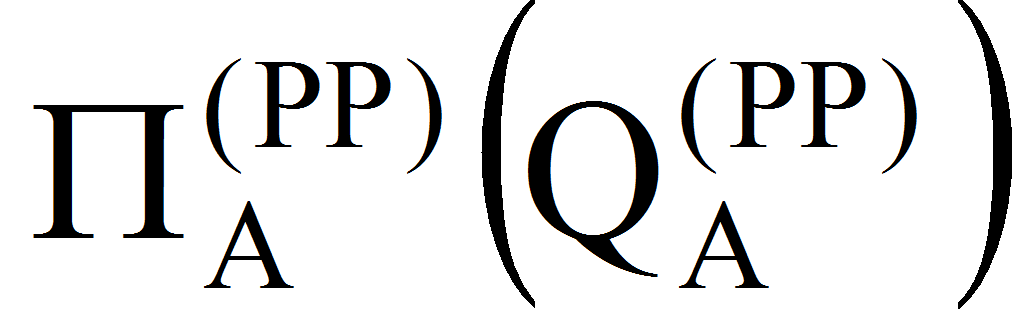=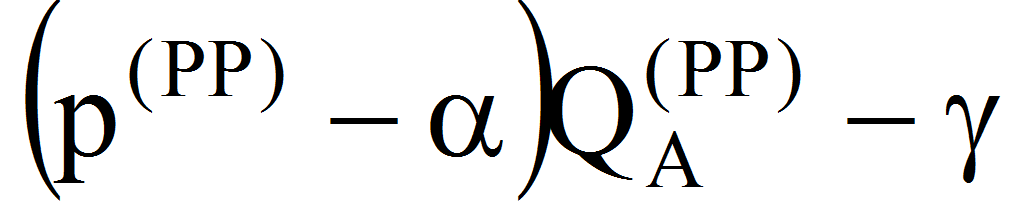=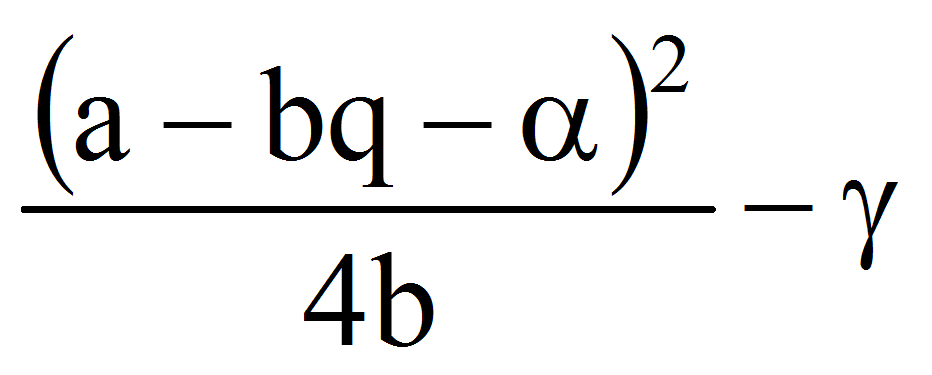respectively: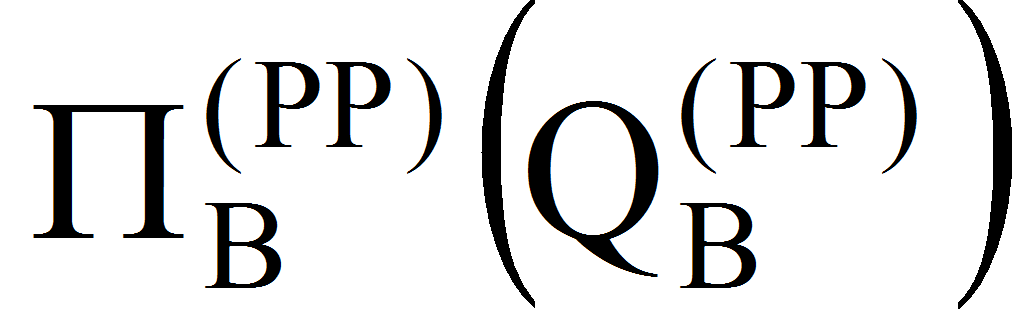=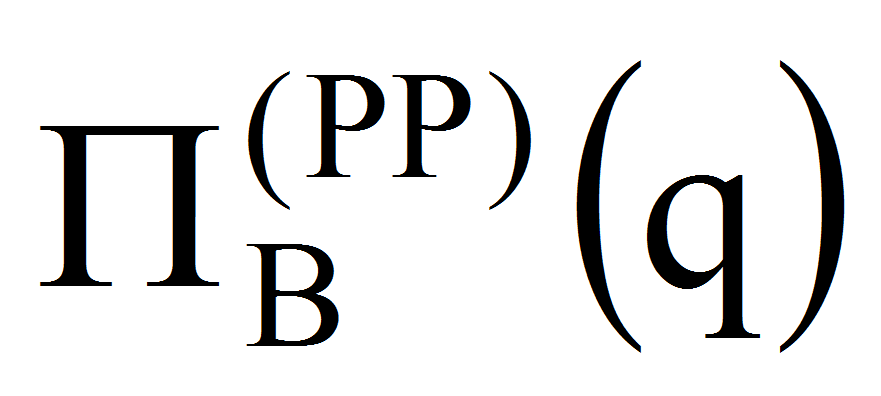=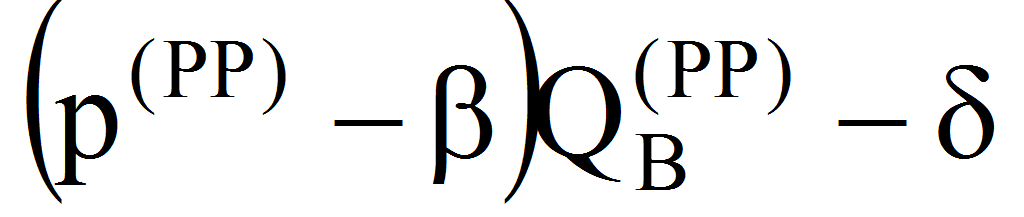=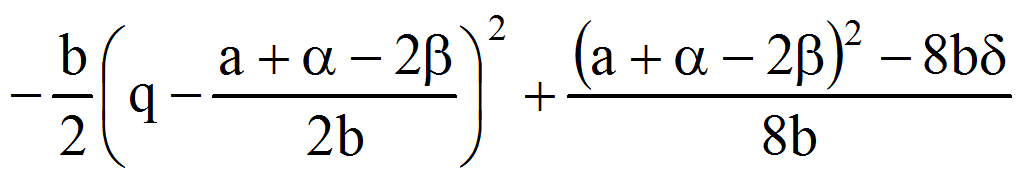Because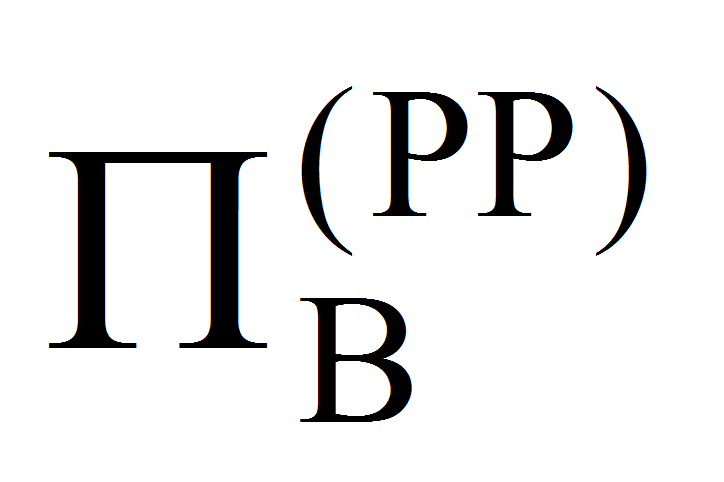is a second degree function in q we have now the following cases:

•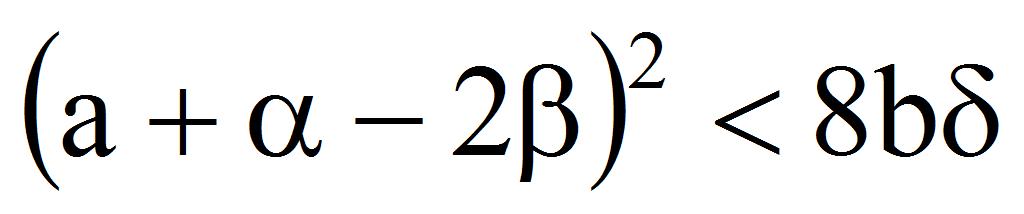implies that